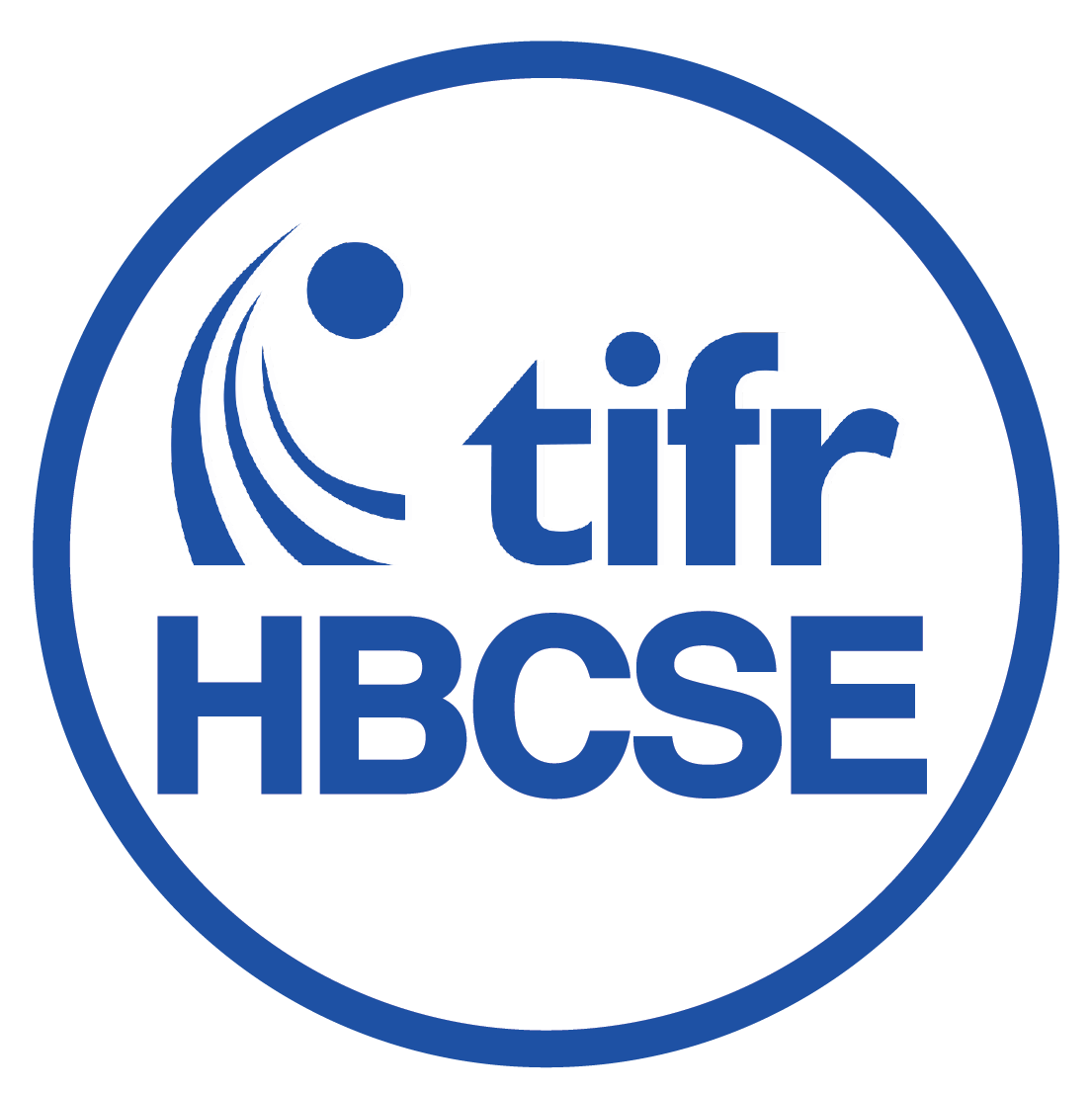This system currently works best on a PC, using Google Chrome

Homi Bhabha Centre for Science Education TATA INSTITUTE OF FUNDAMENTAL RESEARCH# Derivations

### Building a mathematical system that can 'act out' real world changes

The process of derivation 'loads' real world activity into equations. This loading process allows equations to 'act out' the changes in the world, such as bodies moving in a straight line, rotating bodies, oscillating bodies, etc. The equation can thus be thought of as a symbol-based 'machine', which can mimic -- act out -- changes in the real world.

As the symbol-based system can act out changes in the world, it can be used to explore, and predict, the changes in the real world. This makes the symbol-based system very useful, as it allows building and exploring many 'what-if' scenarios, without making (costly) changes to the real world.

- designed by Learning Sciences Research Group and Modeling & Conceptual Change Group$$(MC^{2})$$